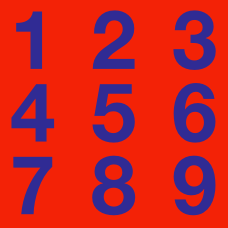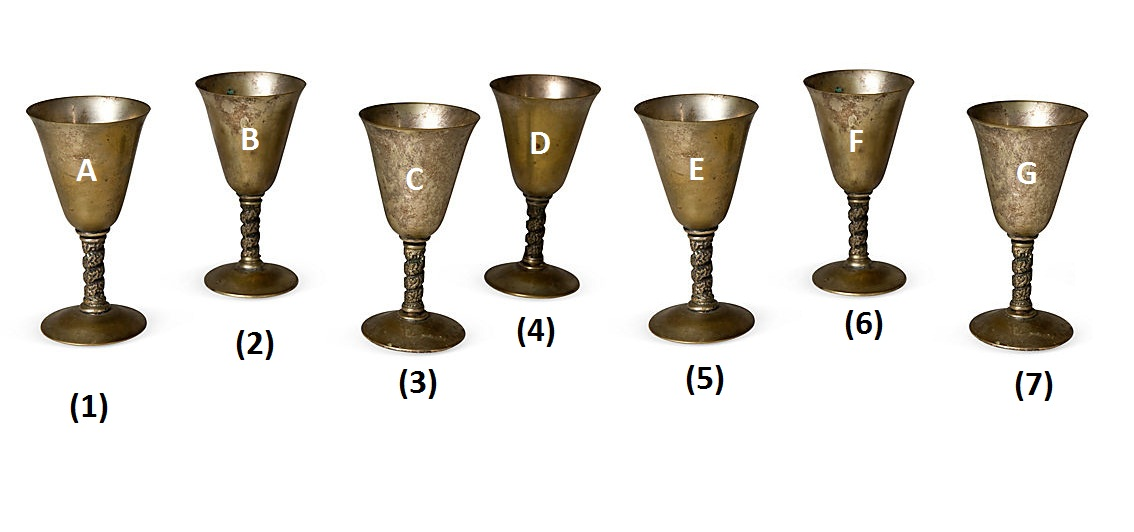Number Theory

# Number Theory Warmups: Level 3 ChallengesOne of the seven goblets above is made of real gold. If you start counting at A and wind back and forth while counting (A, B, C, D, E, F, G, F, E, D, ...), then the golden goblet would be the $1000^\text{th}$ one that you count.

Which one is the golden goblet?

$\Huge {\color{#3D99F6}9}^{{\color{#20A900}8}^{{\color{#D61F06}7}^{{\color{#624F41}6} ^{\color{magenta}5}}}}$

What are the last two digits when this integer fully expanded out?

Find the sum of all positive integers $\displaystyle n$, such that $\displaystyle \dfrac{(n+1)^2}{n+7}$ is an integer.

Find the sum of all prime numbers $p$ that divides $\underset{\text{the digit 1 is repeated }p \text{ times}}{\underbrace{111111111111111\cdots 1}}$.

There is a prime number $p$ such that $16p+1$ is the cube of a positive integer. Find $p$.

×